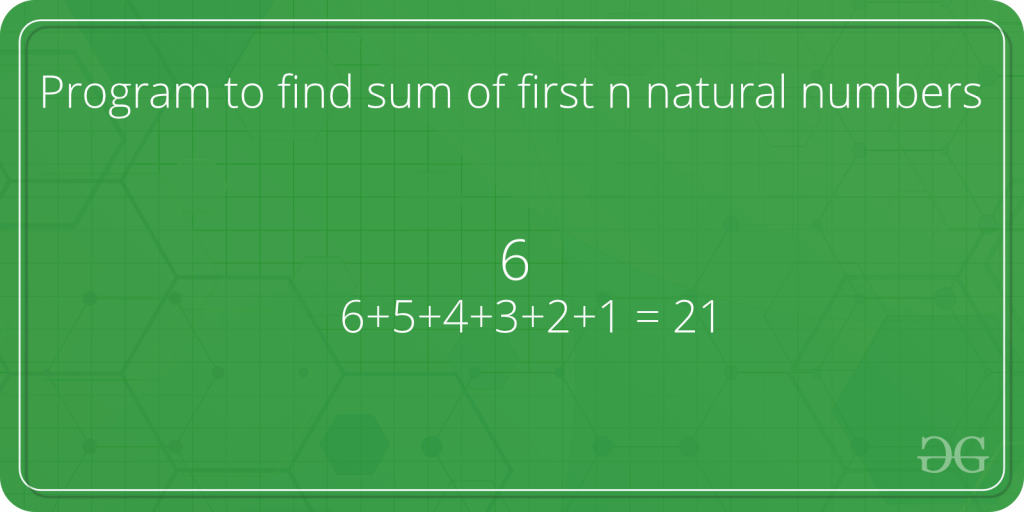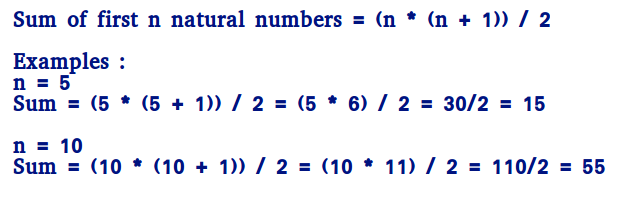Program to find sum of first n natural numbers

• Difficulty Level : Basic
• Last Updated : 08 Apr, 2021

Given a number n, find the sum of first natural numbers.Examples :

Input : n = 3
Output : 6
Explanation :
Note that 1 + 2 + 3 = 6

Input  : 5
Output : 15
Explanation :
Note that 1 + 2 + 3 + 4 + 5 = 15

A simple solution is to do following.

1) Initialize : sum = 0
2) Run a loop from x = 1 to n and
do following in loop.
sum = sum + x

C++

 // CPP program to find sum of first// n natural numbers.#includeusing namespace std; // Returns sum of first n natural// numbersint findSum(int n){   int sum = 0;   for (int x=1; x<=n; x++)     sum = sum + x;   return sum;} // Driver codeint main(){  int n = 5;  cout << findSum(n);  return 0;}

Java

 // JAVA program to find sum of first// n natural numbers.import java.io.*; class GFG{     // Returns sum of first n natural    // numbers    static int findSum(int n)    {        int sum = 0;        for (int x = 1; x <= n; x++)            sum = sum + x;        return sum;    }     // Driver code    public static void main(String args[])    {        int n = 5;        System.out.println(findSum(n));    }} // This code is contributed by Nikita Tiwari.

Python

 # PYTHON program to find sum of first# n natural numbers. # Returns sum of first n natural# numbersdef findSum(n) :    sum = 0    x = 1    while x <=n :        sum = sum + x        x = x + 1    return sum  # Driver code n = 5print findSum(n) # This code is contributed by Nikita Tiwari.

C#

 // C# program to find sum of first// n natural numbers.using System; class GFG{     // Returns sum of first n natural    // numbers    static int findSum(int n)    {        int sum = 0;        for (int x = 1; x <= n; x++)            sum = sum + x;        return sum;    }     // Driver code    public static void Main()    {        int n = 5;        Console.Write(findSum(n));    }} // This code is contributed by vt_m.



Javascript



Output :

15

Time Complexity: O(n)

Auxiliary Space: O(1)
An efficient solution is to use below formula.How does this work?

We can prove this formula using induction.

It is true for n = 1 and n = 2
For n = 1, sum = 1 * (1 + 1)/2 = 1
For n = 2, sum = 2 * (2 + 1)/2 = 3

Let it be true for k = n-1.

Sum of k numbers = (k * (k+1))/2
Putting k = n-1, we get
Sum of k numbers = ((n-1) * (n-1+1))/2
= (n - 1) * n / 2

If we add n, we get,
Sum of n numbers = n + (n - 1) * n / 2
= (2n + n2 - n)/2
= n * (n + 1)/2

C++

 // Efficient CPP program to find sum of first// n natural numbers.#includeusing namespace std; // Returns sum of first n natural// numbersint findSum(int n){   return n * (n + 1) / 2;} // Driver codeint main(){  int n = 5;  cout << findSum(n);  return 0;}

Java

 // Efficient JAVA program to find sum// of first n natural numbers.import java.io.*; class GFG{         // Returns sum of first n natural    // numbers    static int findSum(int n)    {        return n * (n + 1) / 2;    }     // Driver code    public static void main(String args[])    {        int n = 5;        System.out.println(findSum(n));    }} // This code is contributed by Nikita Tiwari.

Python

 # Efficient CPP program to find sum# of first n natural numbers. # Returns sum of first n natural# numbersdef findSum(n) :    return n * (n + 1) / 2     # Driver coden = 5print findSum(n) # This code is contributed by Nikita Tiwari.

C#

 // Efficient C# program to find sum// of first n natural numbers.using System; class GFG{         // Returns sum of first n natural    // numbers    static int findSum(int n)    {        return n * (n + 1) / 2;    }     // Driver code    public static void Main()    {        int n = 5;        Console.Write(findSum(n));    }} // This code is contributed by vt_m.



Javascript



Output:

15

Time Complexity: O(1)

Auxiliary Space: O(1)
The above program causes overflow, even if the result is not beyond integer limit. We can avoid overflow up to some extent by doing division first.

C++

 // Efficient CPP program to find sum of first// n natural numbers that avoids overflow if// result is going to be within limits.#includeusing namespace std; // Returns sum of first n natural// numbersint findSum(int n){   if (n % 2 == 0)      return (n/2) * (n+1);    // If n is odd, (n+1) must be even   else      return  ((n + 1) / 2) * n;} // Driver codeint main(){  int n = 5;  cout << findSum(n);  return 0;}

Java

 // Efficient JAVA program to find sum of first// n natural numbers that avoids overflow if// result is going to be within limits.import java.io.*; class GFG{     // Returns sum of first n natural    // numbers    static int findSum(int n)    {        if (n % 2 == 0)            return (n / 2) * (n + 1);         // If n is odd, (n+1) must be even        else            return ((n + 1) / 2) * n;    }     // Driver code    public static void main(String args[])    {        int n = 5;        System.out.println(findSum(n));    }} //This code is contributed by Nikita Tiwari.

Python

 # Efficient Python program to find the sum # of first n natural numbers that avoid# overflow if the result is going to be# within limits. # Returns sum of first n natural# numbersdef findSum(n) :    if (n % 2 == 0) :        return (n / 2) * (n + 1)     # If n is odd, (n+1) must be even    else :       return  ((n + 1) / 2) * n        # Driver coden = 5print findSum(n) # This code is contributed by Nikita Tiwari.

C#

 // Efficient C# program to find the sum of first// n natural numbers that avoid overflow if// result is going to be within limits.using System; class GFG{     // Returns sum of first n natural    // numbers    static int findSum(int n)    {        if (n % 2 == 0)            return (n / 2) * (n + 1);         // If n is odd, (n+1) must be even        else            return ((n + 1) / 2) * n;    }     // Driver code    public static void Main()    {        int n = 5;        Console.Write(findSum(n));    }} // This code is contributed by vt_m.



Javascript



Output:

15

Time Complexity: O(1)

Auxiliary Space: O(1)
This article is contributed by Karik. If you like GeeksforGeeks and would like to contribute, you can also write an article using contribute.geeksforgeeks.org or mail your article to contribute@geeksforgeeks.org. See your article appearing on the GeeksforGeeks main page and help other Geeks.